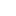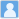• ### Design of 50 MeV proton microbeam based on cyclotron accelerator

分类： 核科学技术 >> 粒子加速器 提交时间： 2023-06-02

摘要：High-energy proton microbeam facilities are powerful tools in space science, biology, and cancer therapy studies. The primary limitations of the 50 MeV proton microbeam system are the poor beam quality provided by the cyclotron and the problem of intense scattering in the slit position. Here, we present an optical design for a cyclotron-based 50 MeV high-energy proton microbeam system with a micron-sized resolution. The microbeam system, which has an Oxford triplet lens configuration, has relatively small spherical aberrations and is insensitive to changes in the beam divergence angle and momentum spread. In addition, the energy filtration included in the system can reduce the beam momentum spread from 1% to 0.02%. The effects of lens parasitic aberrations and the lens fringe field on the beam spot resolution are also discussed. In addition, owing to the severe scattering of 50 MeV protons in slit materials, a slit system model based on the Geant4 toolkit enables the quantitative analysis of scattered protons and secondary particles. For the slit system settings under a 10-micron final beam spot, very few scattered protons can enter the quadrupole lens system and affect the focusing performance of the microbeam system, but the secondary radiation of neutrons and gamma rays generated at the collimation system should be considered for the 50 MeV proton microbeam. These data demonstrate that a 50 MeV proton microbeam system with a micron-sized beam spot based on a cyclotron is feasible.通过
• ### Search for $\psi(4S)\to\eta J/\psi$ in $B^\pm \to \eta J/\psi K^\pm$ and $e^+ e^- \to \eta J/\psi$ processes

分类： 物理学 >> 核物理学 提交时间： 2016-09-13Gao Xu-yang Wang Xiao-long Shen Cheng-ping

摘要：We search for the ψ(4S) state in $B^\pm \ra \eta J/\psi K^\pm$ and $e^+e^- \ra \eta J/\psi$ based on the Belle measurements with the assumed mass M=(4230±8) MeV/c2 and width Γ=(38±12) MeV. No significant signal is observed in the ηJ/ψ mass spectra. The 90\% confidence level upper limit on the product branching fraction $\BR(B^\pm \ra \psi(4S)K^\pm)\BR(\psi(4S) \ra \eta J/\psi)<6.8\times 10^{-6}$ is obtained in$B^\pm \ra \eta J/\psi K^\pm$ decays. By assuming the partial width of ψ(4S)→e+e− being 0.63 keV, the branching fraction limit $\BR(\psi(4S) \ra \eta J/\psi) < 1.3\%$ is obtained at the 90\% confidence level in$e^+e^- \ra \eta J/\psi$, which is consistent with the theoretical prediction.

同行评议状态:待评议